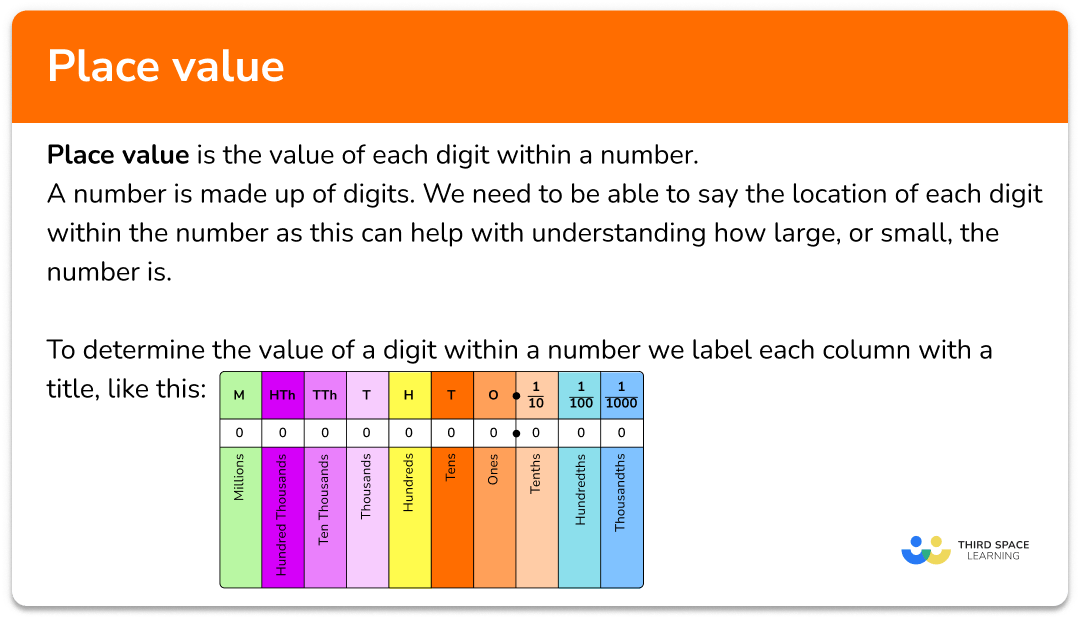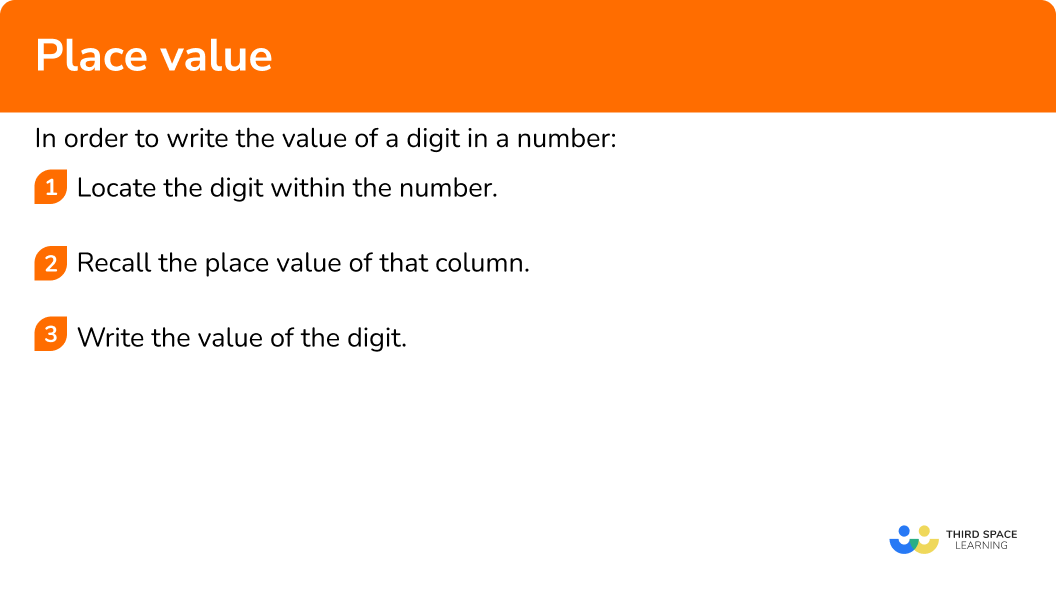# Place Value

Here we will learn about place value, including how to use it for integers, decimals and measures.

There are also place value worksheets based on Edexcel, AQA and OCR exam questions, along with further guidance on where to go next if you’re still stuck.

## What is place value?

Place value is the value of each digit within a number.

A number is made up of digits, for example the two-digit number 54 (fifty four), has two digits, 5 and 4. We need to be able to say the value of each digit within the number as this can help with understanding how large or small the number is and can help us to order numbers.

To determine the value of a digit within a number we use a place value chart.

For example, the number 54 would look like this, where the 4 is in the ones column, and the 5 is in the tens column.

This means that the number 54 is equivalent to 5 tens and 4 ones.

5 tens is the same as 5\times{10}=50 and 4 ones is the same as 4\times{1}=4.

Adding the two values of 50 and 4 gives us the number 54.

To write the place value of a digit within a number, we must know the column that the digit is sitting in. We determine this by lining up the ones column first, and then we write every other digit in the correct column next to it.

### What is place value?### Integer place value

Here we will consider the place value of digits to the left of the decimal point.

• Using a comma or a space

The comma or space is used to help the reader to read a number efficiently. They are used for the digits to the left of the decimal point, separating 3 digits at a time.

For example,

The number 9456382 is easier to read by placing a space after the millions digit (9), and a space after the thousands digit (6). This would give us the number 9 \ 456 \ 382 which would read as,

nine million, four hundred and fifty six thousand, three hundred and eighty two.

• Zero place holder

If the first digit of a number is to the left of the decimal point (ones, ten thousands, millions etc), we do not need to write a zero before the start of the number.

This is because, for example. the number 293 is exactly the same as the number 000293 and so the zeros before the digit 2 in the hundreds column are unnecessary.

However, if the value within the column is 0, we must write a zero within that place value.

For example,  5084 is the number five thousand and eighty four.

If we missed the 0 out of the number, we would get the number 584, read as five hundred and eighty four, which is a different number.

• Larger numbers

We can use place value to represent very large numbers.

### Decimal place value

Here we are considering the place value of digits to the right of the decimal point.

Decimals are read digit by digit.

For example, the decimal number 12.34 would be read as twelve point three four, and not twelve point thirty four. This is the reason why we do not separate the decimals using a comma or a space.

For small numbers that contain a lot of zeros such as 0.003, we would pronounce this number as zero point zero zero three.

• Zero place holder

For decimals, we must write a 0 placeholder in any column between the decimal point and the first non-zero digit to the right of the decimal point. If there are no ones, we must also write a zero in the ones column.

For example, the number four thousandths is written as 0.004 which has a 0 place holder in the ones, tenths and hundredths columns.

• Very small numbers

We can use place value to represent very small numbers.

## How to write the value of a digit in a number

In order to write the value of a digit in a number:

1. Locate the digit within the number.
2. Recall the place value of that column.
3. Write the value of the digit.

### Explain how to write the value of a digit in a number## Place value examples

### Example 1: ones

Write down the value of the digit 3 in the four-digit number 4163.

1. Locate the digit within the number.

2Recall the place value of that column.

The 3 is in the ones column.

3Write the value of the digit.

The value of the digit 3 in the number 4163 is 3 ones, or 3.

### Example 2: hundreds

Write down the value of the digit 4 in the number 385,431.

The 4 is in the hundreds column.

The value of the digit 4 in the number 385431 is four hundred, or 400.

### Example 3: ten thousands

Write down the value of the digit 2 in the number 1,025,634.

The 2 is in the ten thousands column.

The value of the digit 2 in the number 1,025,634 is twenty thousand, or 20,000.

### Example 4: tenths

Write down the value of the digit 2 in the number 3.264.

The 2 is in the tenths column.

The value of the digit 2 in the number 3.264 is two tenths, or 0.2.

### Example 5: hundredths

Write down the value of the digit 7 in the number 0.0783.

The 7 is in the hundredths column.

The value of the digit 7 in the number 0.0783 is seven hundredths, or 0.07.

### Example 6: thousandths

Write down the value of the digit 4 in the number 10.05401.

The 4 is in the thousandths column.

The value of the digit 4 in the number 10.05401 is four thousandths, or 0.004.

### Common misconceptions

• Thousands or thousandths

Numbers to the right of the decimal point are parts of a whole and so they each end in ths. Numbers to the left of the decimal point do not end in ths as they are whole numbers.

• Including the digits after the required digit

All of the digits after the required place value are sometimes wrongly included, for example the value of 4 in the number 243 is given as forty three, or 43.

The correct solution is forty, or 40.

• Including a ones column

The first column to the right of the decimal point is the tenths column – there is no ones column.

For example, the value of the digit 2 in the number 3.524 may be given as two tenths or 0.02 which is incorrect. The correct answer is two hundredths, or 0.02.

Place value is part of our series of lessons to support revision on rounding numbers, and upper and lower bounds. You may find it helpful to start with the main rounding numbers lesson for a summary of what to expect, or use the step by step guides below for further detail on individual topics. Other lessons in this series include:

### Practice place value questions

1. Write the value of 2 in the number 475,321.

21202Two hundredsThe digit 2 is in the tens column.

2. Write the value of 1 in the number 1,000,253.

1,0001000001,000,000One hundred thousandThe digit 1 is in the millions column.

3. Write the value of 3 in the number 483,201.

3,00030010,000Ten thousandThe digit 3 is in the thousands column.

4. Write the value of 7 in the number 6.072.

Seven hundredthsSeven hundredsSeven tenthsSeven tensThe digit 7 is in the hundredths column.

5. Write the value of 8 in the number 0.0008.

Eight thousandthsEighty hundredEight ten thousandthsEight tenthsThe digit 8 is in the ten thousandths column.

6. Write the value of 4 in the number 4.825.

44000Four thousandths0.4The digit 4 is in the ones column.

### Place value GCSE questions

1. (a) Write in figures the number fifty seven thousand and four.

(b) Write in words the number 0.052.

(c) Write the value of the digit 4 in the number 5,846,732.

(3 marks)

(a) 57,004

(1)

(b) Zero point zero five two

(1)

(c) 40,000 or forty thousand

(1)

2. (a) Calculate 0.43 \div 100.

(b) State the value of the digit from your answer to part (a) that is in the thousandths column.

(2 marks)

(a) 0.0043

(1)

(b) 4 thousandths or 0.004

(1)

3. (a) Dom says

“The value of the digit 3 in the number 0.7534 is 3000 ”.

Is Dom correct?

(b) The record for a bobsleigh run on a track is 16.543 seconds. The record is beaten by 2 thousandths of a second.

What is the new record for the track?

(5 marks)

(a)

No

(1)

Dom has stated that the 3 is in the thousands column whereas it is in the thousandths column (part of an integer) or 0.003 .

(1)

(b)

0.002

(1)

16.543-0.002

(1)

16.541

(1)

## Learning checklist

You have now learned how to:

• Understand and use place value for decimals, measures and integers of any size

## Still stuck?

Prepare your KS4 students for maths GCSEs success with Third Space Learning. Weekly online one to one GCSE maths revision lessons delivered by expert maths tutors.

Find out more about our GCSE maths tuition programme.